## Thursday, May 14, 2015

### About how ace(...,marginal=TRUE) does not compute marginal ancestral states [but ace(...,marginal=FALSE) does]

Just a quick note on this issue. The function `ace` for `type="discrete"` previously suffered from the issue that it did not (as many people nonetheless at the time believed) compute marginal ancestral states - but instead conditional probabilities based only on the information contained in the subtree descended from each node.

Thankfully, this has now been fixed - but the documentation of the function `ace` (as well as the unfortunate choice of argument names) still makes things much more confusing, in my opinion, then they need to be.

To summarize the main point of this post: `ace(...,marginal=TRUE)` does not compute marginal ancestral states - rather `ace(...,marginal=FALSE)` (the default) does.

In the documentation of `ace`, the argument `marginal` is defined in the following way:

`marginal`   a logical (relevant if `type = "d"`). By default, the joint reconstruction of the ancestral states are done. Set this option to `TRUE` if you want the marginal reconstruction (see details.)”

“If `marginal = TRUE`, a marginal estimation procedure is used (this was the only choice until ape 3.1-1). With this second method, the likelihood values at a given node are computed using only the information from the tips (and branches) descending from this node. With the joint estimation, all information is used for each node.”

This gives us a hint that `marginal=TRUE` may not in fact be returning the marginal reconstruction - because the marginal ancestral states do not use information solely from the subtree descended from the node, rather marginal reconstruction asks (node by node) what is the most likely state for this node, integrating over all the possible states, over all the other nodes in the tree, in proportion to their probability. This differs from joint reconstruction not in using only a subset of the data (as implied) but in that joint reconstruction asks instead what is the set of states at all nodes with the highest likelihood. This set of states may or may not be the set of states obtained using marginal reconstruction (or, similarly, the set of states that individually have the highest scaled likelihoods / empirical Bayes posterior proabilities).

That `ace(...,marginal=TRUE)` does not give the marginal reconstruction (and, conversely, that `ace(...,marginal=FALSE)`, the default, does give the marginal reconstructions) can be shown by comparison to other functions that do compute these reconstructions as follows:

``````## first simulate data & tree
tree<-pbtree(n=26,tip.label=LETTERS,scale=1)
Q<-matrix(c(-1,1,0,1,-2,1,0,1,-1),3,3)
rownames(Q)<-colnames(Q)<-letters[1:3]
tree<-sim.history(tree,Q)
``````
``````## Done simulation(s).
``````
``````x<-tree\$states
x
``````
``````##   A   B   C   D   E   F   G   H   I   J   K   L   M   N   O   P   Q   R
## "b" "a" "c" "b" "c" "c" "b" "b" "a" "a" "b" "a" "b" "b" "b" "c" "c" "b"
##   S   T   U   V   W   X   Y   Z
## "a" "b" "a" "a" "a" "a" "a" "a"
``````

Start with `ace` for `marginal=TRUE` & `marginal=FALSE`:

``````fit.ace.true<-ace(x,tree,type="discrete",model="SYM",marginal=TRUE)
fit.ace.false<-ace(x,tree,type="discrete",model="SYM",marginal=FALSE)
plotTree(tree,mar=c(0.1,0.1,5.1,0.1),offset=0.5)
nodelabels(pie=fit.ace.true\$lik.anc,cex=0.7)
tiplabels(pie=to.matrix(x,seq=letters[1:3]),cex=0.5)
title(main="ace(...,marginal=TRUE)")
``````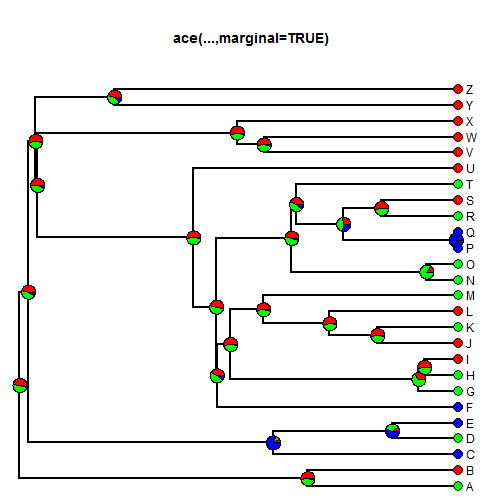``````plotTree(tree,mar=c(0.1,0.1,5.1,0.1),offset=0.5)
nodelabels(pie=fit.ace.false\$lik.anc,cex=0.7)
tiplabels(pie=to.matrix(x,seq=letters[1:3]),cex=0.5)
title(main="ace(...,marginal=FALSE)")
``````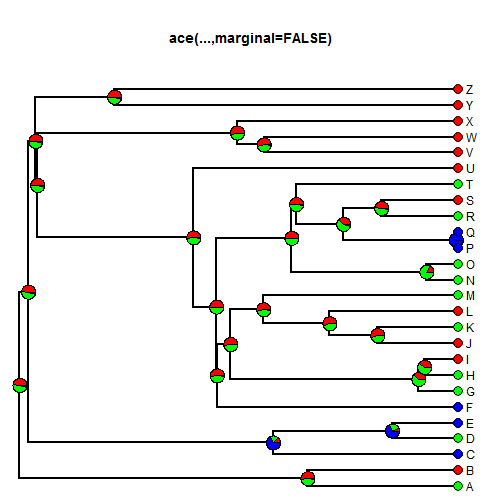``````plot(fit.ace.true\$lik.anc,fit.ace.false\$lik.anc,
xlab="ace(...,marginal=TRUE)",ylab="ace(...,marginal=FALSE)")
``````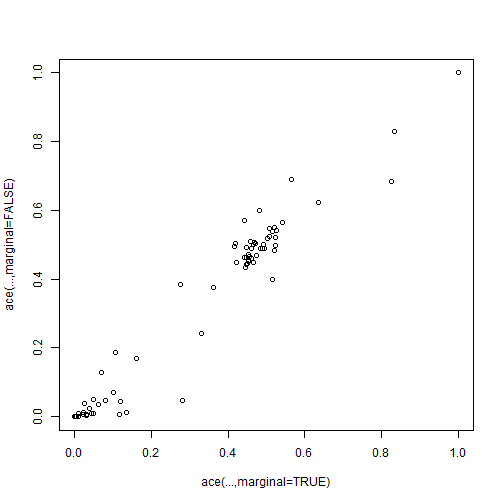Now we can compare to marginal ancestral state reconstruction implemented in other functions. In phytools, I have the function `rerootingMethod` (details here) that takes advantage of the re-rooting algorithm of Yang et al.:

``````fit.rrm<-rerootingMethod(tree,x,model="SYM")
plotTree(tree,mar=c(0.1,0.1,5.1,0.1),offset=0.5)
nodelabels(pie=fit.rrm\$marginal.anc,cex=0.7)
tiplabels(pie=to.matrix(x,seq=letters[1:3]),cex=0.5)
title(main="rerootingMethod")
``````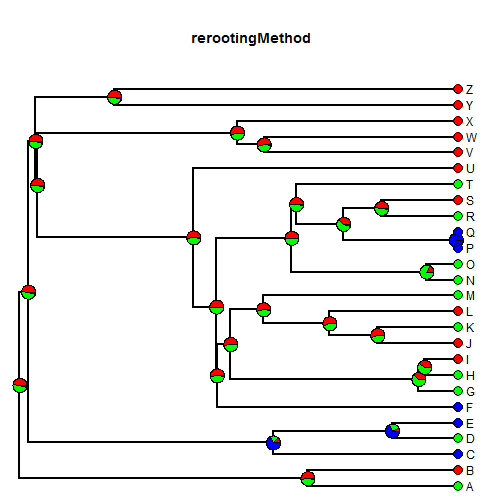We can compare this to `ace(...,marginal=FALSE)`:

``````plot(fit.ace.false\$lik.anc,fit.rrm\$marginal.anc,
xlab="ace(...,marginal=FALSE)",ylab="rerootingMethod")
``````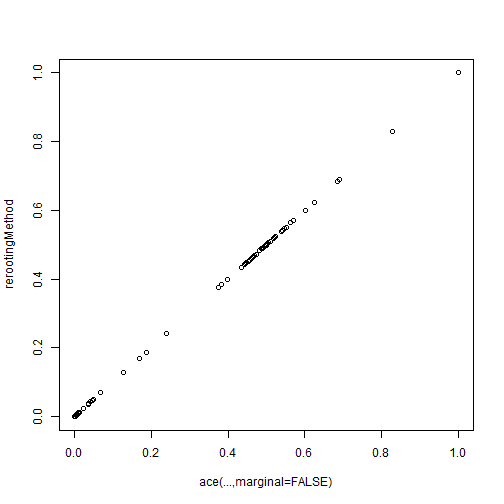Finally, we can use Klaus Schliep's phangorn package as follows (code snippet provided here by Klaus):

``````library(phangorn)
X<-phyDat(as.matrix(x),type="USER",levels=c("a","b","c"))
fit<-pml(tree,X)
fit<-optim.pml(fit,optEdge=FALSE,optRate=TRUE,optQ=TRUE)
``````
``````## optimize rate matrix:  -30.36139 --> -27.84751
## optimize rate:  -27.84751 --> -26.61064
## optimize rate matrix:  -26.61064 --> -26.40027
## optimize rate:  -26.40027 --> -26.32162
## optimize rate matrix:  -26.32162 --> -26.27694
## optimize rate:  -26.27694 --> -26.2545
## optimize rate matrix:  -26.2545 --> -26.24114
## optimize rate:  -26.24114 --> -26.23345
## optimize rate matrix:  -26.23345 --> -26.2286
## optimize rate:  -26.2286 --> -26.22557
## optimize rate matrix:  -26.22557 --> -26.22358
## optimize rate:  -26.22358 --> -26.22228
## optimize rate matrix:  -26.22228 --> -26.22139
## optimize rate:  -26.22139 --> -26.22079
## optimize rate matrix:  -26.22079 --> -26.22038
## optimize rate:  -26.22038 --> -26.22009
## optimize rate matrix:  -26.22009 --> -26.21989
## optimize rate:  -26.21989 --> -26.21975
## optimize rate matrix:  -26.21975 --> -26.21965
## optimize rate:  -26.21965 --> -26.21957
``````
``````fit.phangorn<-ancestral.pml(fit)
anc.ml<-t(sapply(1:tree\$Nnode+Ntip(tree),function(i,x) x[[as.character(i)]][1,],x=fit.phangorn))
plotTree(tree,mar=c(0.1,0.1,5.1,0.1),offset=0.5)
nodelabels(pie=anc.ml,cex=0.7)
tiplabels(pie=to.matrix(x,seq=letters[1:3]),cex=0.5)
title(main="phangorn::ancestral.pml")
``````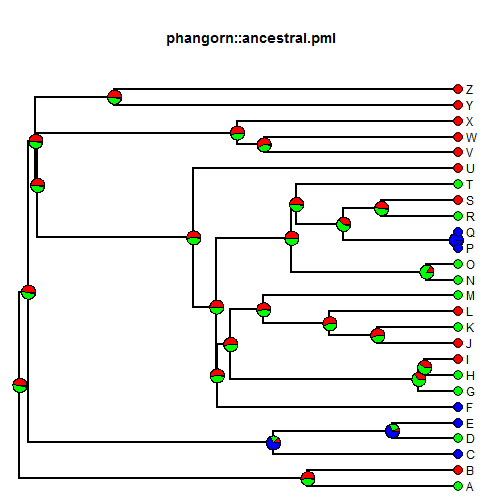``````plot(fit.ace.false\$lik.anc,anc.ml,xlab="ace(...,marginal=FALSE)",
ylab="phangorn::ancestral.pml")
``````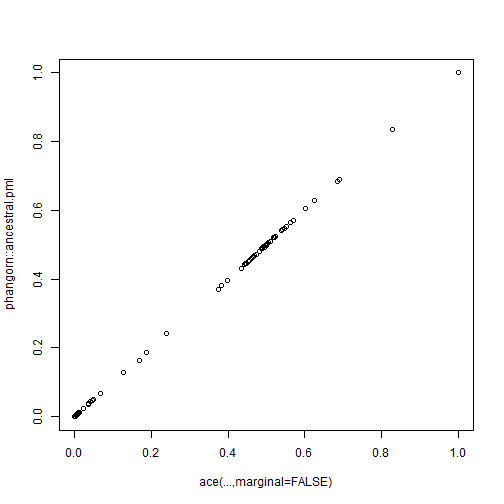OK, well I guess that the point of this lengthy exercise is not to criticize ace, or ape (which is an incredible, impressive, multifunctional package at the core of most phylogenetics in R), but merely to point out that ace may not be doing exactly what you think it's doing - when you'd think it would be reasonable for it to be doing that thing. The good news is that with the default settings in recent versions of ape the reconstructed ancestral states returned are marginal ancestral states (aka. empirical Bayesian posterior probabilities), which is probably what we should be using for ancestral state reconstruction of discrete traits on the tree.

#### 1 comment:

1.Update: I sent this post to Emmanuel Paradis & he has informed me that the documentation will be fixed/clarified in future versions of ape.

Note: due to the very large amount of spam, all comments are now automatically submitted for moderation.